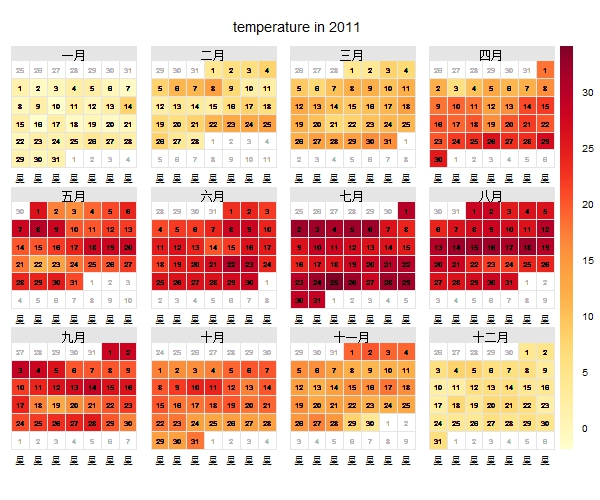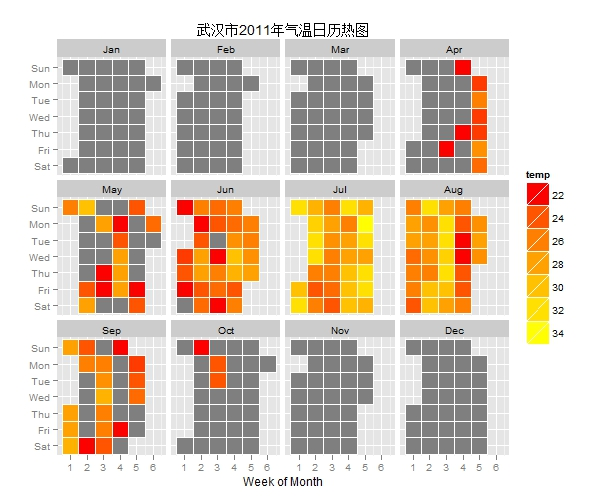看起来不错，但是我们还没完。我们希望挑选出平均温度在22度以上的日子，突出显示出来。所以我们采用第三种方法，用ggplot2包来绘制日历热图，图形显示如下（参考了MarginTale的这篇文章）。上图数日子是竖着来数的，横轴表示每月的第几周，纵轴表示星期几。灰色部分表示当天平均温度在22度以下，有色彩的区块表示在22度以上。颜色越偏黄则表示温度越高。在2011年，立夏的时间是5月6日，立秋是8月8日，但可以观察到立秋之后仍有很多日子的平均温度在22度以上。这就是我们所俗称的“秋老虎”。如果按照气候学的定义，四月末就有五天以上连续的高温天气，照这样看夏天应该在四月末就开始了，一直延续到十月初结束。而天文学上的夏季则是六、七、八三个月，看到这三个月基本上全是22度以上，而且高温天气集中在七八两个月，这也正是学校放暑假的时间段。这样看来，似乎天文学的夏季定义是比较符合我们人体的感觉的。其它的要么偏短，要么偏长。

（最后要说的是，本人并非气象专家，本文也没有考虑到湿度对体感温度的影响，或是其它因素。主要还是向各位介绍R语言中日历热图的绘制以及数据的获取。）

# 加载所需扩展包
library(RCurl)
library(RJSONIO)
require(quantmod)
library(ggplot2)

# 提取武汉市2011年一年的历史数据
date<- seq.Date(from=as.Date('2011-01-01'),
to=as.Date('2011-12-31'), by='1 day')
date.range<- as.character(format(date,"%Y%m%d"))
n <- length(date.range)
temp <- humi <- rep(0,n)
for (i in 1:n) {
# 你要用自己申请的API key来代替程序中的yourkey
url <- 'http://api.wunderground.com/api/yourkey/'
finalurl <- paste(url,'history_',date.range[i],
'/q/wuhan.json',sep='')
web <- getURL(finalurl)
raw <-fromJSON(web)
temp[i] <- raw$history$dailysummary[]$meantempm humi[i] <- raw$history$dailysummary[]$humidity
# 在循环内增加一个7秒的暂停，避免连接断开。
Sys.sleep(7)
}
# 将获得的数据整合为数据框，并将温度和湿度转为数值格式
dataset <- data.frame(temp,humi,date,stringsAsFactors=F)
dataset$temp <- as.numeric(dataset$temp)
dataset$humi <- as.numeric(dataset$humi)

# 用openair包绘制日历热图
install.packages('openair')
library(openair)
calendarPlot(dataset,pollutant='temp',year=2011)

# 用ggplot2包绘制日历热图
# 复制一个新的数据框
dat <- dataset
# 先取得月份，再转为因子格式
dat$month<-as.numeric(as.POSIXlt(dat$date)$mon+1) dat$monthf<-factor(dat$month,levels=as.character(1:12), labels=c("Jan","Feb","Mar","Apr","May","Jun","Jul", "Aug","Sep","Oct","Nov","Dec"),ordered=TRUE) # 得到每周的星期，也转为因子格式 dat$weekday = as.POSIXlt(dat$date)$wday
dat$weekdayf<-factor(dat$weekday,levels=rev(0:6),
labels=rev(c("Sun","Mon","Tue","Wed","Thu","Fri","Sat")),ordered=TRUE)
# 先得到全年的周序号，然后得到每个月的周序号
dat$week <- as.numeric(format(dat$date,"%W"))
dat<-ddply(dat,.(monthf),transform,monthweek=1+week-min(week))
# 绘图
P <- ggplot(dat, aes(monthweek, weekdayf, fill = temp)) +
geom_tile(colour='white') +
facet_wrap(~monthf ,nrow=3) +